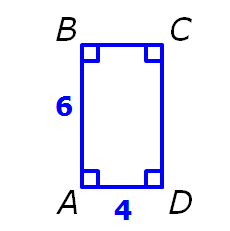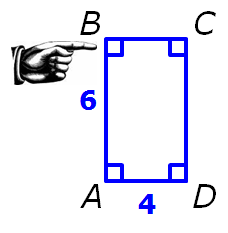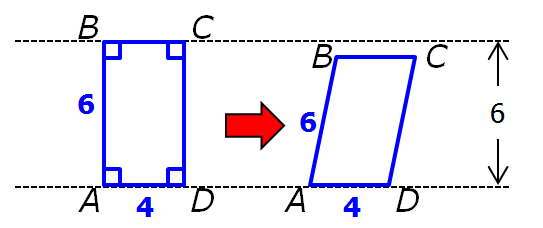It is currently 26 May 2019, 19:31### GMAT Club Daily Prep

#### Thank you for using the timer - this advanced tool can estimate your performance and suggest more practice questions. We have subscribed you to Daily Prep Questions via email.

Customized
for You

we will pick new questions that match your level based on your Timer History

Track
Your Progress

every week, we’ll send you an estimated GMAT score based on your performance

Practice
Pays

we will pick new questions that match your level based on your Timer History

#### Not interested in getting valuable practice questions and articles delivered to your email? No problem, unsubscribe here.# The area of region ABCDQuestion banks Downloads My Bookmarks Reviews Important topics
Author Message
TAGS:
FounderJoined: 18 Apr 2015
Posts: 6647
Followers: 107

Kudos [?]: 1275 , given: 6017

The area of region ABCD [#permalink]
Expert's post00:00

Question Stats:63% (00:41) correct36% (00:36) wrongbased on 22 sessions
Attachment:#GREpracticequestion ABCD is a parallelogram.jpg [ 5.26 KiB | Viewed 949 times ]

ABCD is a parallelogram

 Quantity A Quantity B The area of the region ABCD 24

A)The quantity in Column A is greater.
B)The quantity in Column B is greater.
C)The two quantities are equal.
D)The relationship cannot be determined from the information given.
[Reveal] Spoiler: OA

_________________GRE InstructorJoined: 10 Apr 2015
Posts: 1802
Followers: 58

Kudos [?]: 1686  , given: 8

Re: The area of region ABCD [#permalink]
3
This post received
KUDOS
Expert's post
Carcass wrote:ABCD is a parallelogram

 Quantity A Quantity B The area of the region ABCD 24

First notice that IF a RECTANGLE had lengths the same as the parallelogram......then the area of that rectangle would be 24 (since area = base x height = 4x6 = 24)

However, the given quadrilateral is NOT a rectangle.
Instead, the rectangle has been "pushed" over.Notice that if we "push" the rectangle far enough, then its measurements will be the same as in the given diagram/Most importantly, the height is now less than 6
The area of a parallelogram = base x height
So, the area = 4 x (some number that's less than 6) = some value that's less than 24

ASIDE: We need not find the actual height of the parallelogram; we need only recognize that it will be less than 6.

We have:
Quantity A: some value that's less than 24
Quantity B: 24

Answer: B
_________________

Brent Hanneson – Creator of greenlighttestprep.comSign up for my free GRE Question of the Day emails

GRE Forum Moderator
Joined: 29 May 2018
Posts: 125
Followers: 0

Kudos [?]: 86 , given: 4

Re: The area of region ABCD [#permalink]
Carcass..if not above logic , what could be the other solution.
FounderJoined: 18 Apr 2015
Posts: 6647
Followers: 107

Kudos [?]: 1275 , given: 6017

Re: The area of region ABCD [#permalink]
Expert's post
Sorry, In this particular case your geometry notions applied to the figure would lead to the right answer pretty fast.

I do not see any other strategy such as picking numbers, not here at least.

Regards
_________________
FounderJoined: 18 Apr 2015
Posts: 6647
Followers: 107

Kudos [?]: 1275 , given: 6017

Re: The area of region ABCD [#permalink]
Expert's post
Sorry, In this particular case your geometry notions applied to the figure would lead to the right answer pretty fast.

I do not see any other strategy such as picking numbers, not here at least.

Regards
_________________
Supreme Moderator
Joined: 01 Nov 2017
Posts: 370
Followers: 5

Kudos [?]: 111 , given: 4

Re: The area of region ABCD [#permalink]
Expert's post
msk0657 wrote:
Carcass..if not above logic , what could be the other solution.

see what can be the area of the given parallelogram..
The area of a parallelogram is Base * Height.
Base is 4 and height is a, which will be less than 6 as 6 is the hypotenuse and thus he largest side of right angled triangle.
therefore area will be < 4*6 or <24.
hence B>A.

B
Attachments001.png [ 44.36 KiB | Viewed 1307 times ]

_________________

Some useful Theory.
1. Arithmetic and Geometric progressions : https://greprepclub.com/forum/progressions-arithmetic-geometric-and-harmonic-11574.html#p27048
2. Effect of Arithmetic Operations on fraction : https://greprepclub.com/forum/effects-of-arithmetic-operations-on-fractions-11573.html?sid=d570445335a783891cd4d48a17db9825
3. Remainders : https://greprepclub.com/forum/remainders-what-you-should-know-11524.html
4. Number properties : https://greprepclub.com/forum/number-property-all-you-require-11518.html
5. Absolute Modulus and Inequalities : https://greprepclub.com/forum/absolute-modulus-a-better-understanding-11281.html

InternJoined: 05 Dec 2018
Posts: 9
Followers: 0

Kudos [?]: 0 , given: 0

Re: The area of region ABCD [#permalink]
It's quite simple.
The area of a parallelogram will be 6x4xSin125. That's 24Sin125. The value of Sin125 should be less than 1. That will reduce the area below 24. So 24 is more than 24 Sin125.
It's BGRE InstructorJoined: 10 Apr 2015
Posts: 1802
Followers: 58

Kudos [?]: 1686  , given: 8

Re: The area of region ABCD [#permalink]
1
This post received
KUDOS
Expert's post
Carcass wrote:
Attachment:
#GREpracticequestion ABCD is a parallelogram.jpg

ABCD is a parallelogram

 Quantity A Quantity B The area of the region ABCD 24

A)The quantity in Column A is greater.
B)The quantity in Column B is greater.
C)The two quantities are equal.
D)The relationship cannot be determined from the information given.

Here's a different approach:

The area of a parallelogram = (base)(height)

We know the base has length 4......however, we don't know the height:However, if we recognize that we have a RIGHT TRIANGLE hiding in our diagram...... we can use the fact that the hypotenuse is always the longest side in a RIGHT TRIANGLE.
Since the hypotenuse in the above triangle has length 6, we know that the other sides of the right triangle are LESS THAN 6

This means the triangle's height is less than 6It also means the height of the parallelogram is less than 6

We're now ready to answer the question.

The area of a parallelogram = (base)(height)
= (4)(some number less than 6)
= some number less than 24

So, we get:
QUANTITY A: some number less than 24
QUANTITY B: 24

Answer: B

RELATED VIDEO FROM MY COURSE

_________________

Brent Hanneson – Creator of greenlighttestprep.comSign up for my free GRE Question of the Day emailsRe: The area of region ABCD   [#permalink] 14 Feb 2019, 12:46
Display posts from previous: Sort by

# The area of region ABCDQuestion banks Downloads My Bookmarks Reviews Important topicsPowered by phpBB © phpBB Group Kindly note that the GRE® test is a registered trademark of the Educational Testing Service®, and this site has neither been reviewed nor endorsed by ETS®.